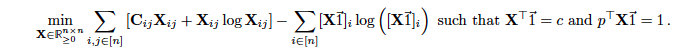# Unable to implement this using entr() function. Any suggestions on how to implement this problem on cvx?I believe the essence of the situation is your need to express the following construct in CVX:
`x1*log(x1)+x2*log(x2) - (x1+x2)*log(x1+x2)`

This can be re-written as

``````   x1*(log(x1) - log(x1+x2)) + x2*(log(x2) - log(x1+x2)) =
x1*log(x1/(x1+x2)) + x2*log(x2/(x1+x2)) =
rel_entr(x1,x1+x2) + rel_entr(x2,x1+x2)
``````

I’ll let you extend this in the obvious way to your matrix variable and summation.

Thank you for your quick response and your great help! I will try to use this now. Thanks again !